# Simple UNO calculator

Make simple math with arduino UNO

BeginnerFull instructions provided18,178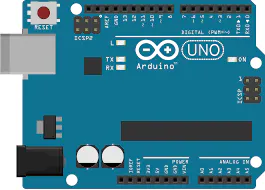## Things used in this project

### Hardware componentsArduino UNO & Genuino UNO Just the board will do it, no need for more. Also, if 'im not mistaken, this will work with any board, original or not. If it works with Arduino IDE, this project works on it.
×1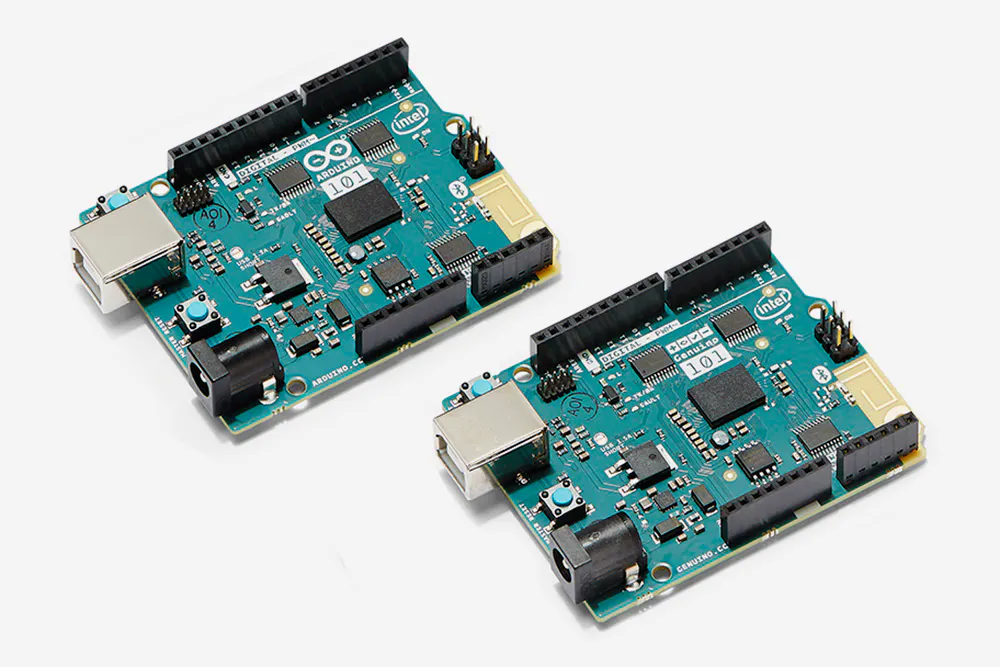Arduino 101 & Genuino 101
×1Arduino Nano R3
×1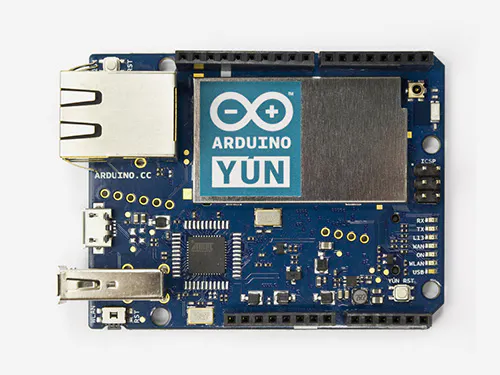Arduino Yun
×1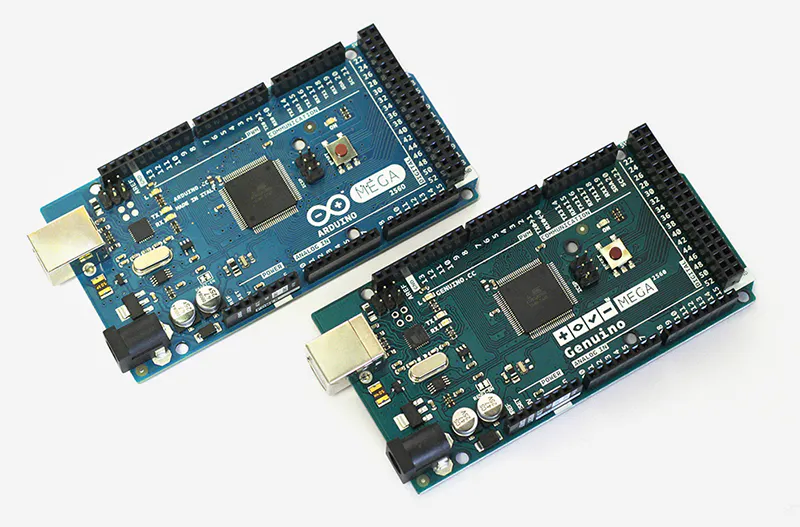Arduino Mega 2560 & Genuino Mega 2560
×1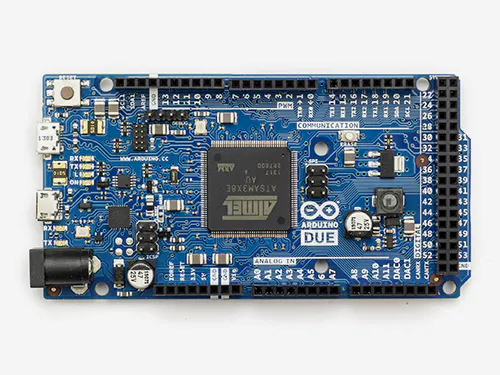Arduino Due
×1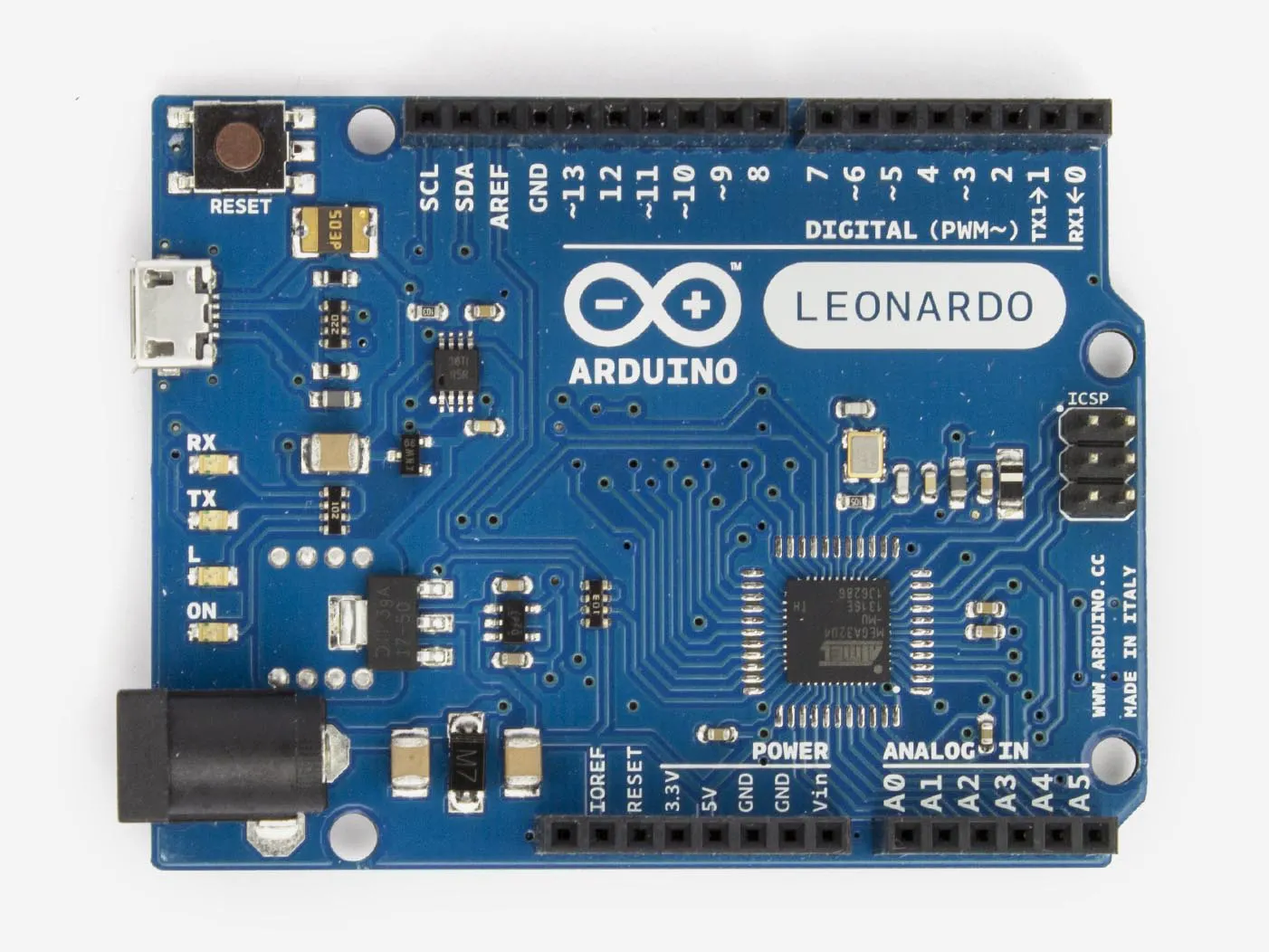Arduino Leonardo
×1

### Software apps and online servicesArduino IDE

## Schematics

### What you need for it to work

Basicaly, just the board is needed, actually## Code

### CODE FOR CALCULATOR

Arduino
You enter the numbers and the signal togheter, like "2+3", or 8*2, and it gives the result
```/* Serial arduino calculator
in this project, you can make basic
arithmetic with the help of arduino,
almost like a very rustic calculator.
It accepts two numbers and a signal, and
makes the operation, witch can be of +, -, * or /.
E.G. : send "2+3" (Without quotes and with no
space separing the info), and arduino answers 5.
Digit "7-3" and arduino te responde com 4.
Criado por João Paulo Rodrigues Poltronieri

This code is on public domain
*/

// first of all, create variables to store
// the information sent to arduino

lng number1; // first number of the calculation,
// sent through the Serial monitor

// If you take a look, it's a long varible, so
// we're able to use big numbers

long number2; // second number sent through the SM

char calSignal; // create a char variable to store
// the calcuation signal.

long result; // result of the calculation

void setup() {
Serial.begin(9600); // begins serial communications
Serial.println("Send me a calculation");
Serial.println("E.G. : 2+3");
Serial.println();
// prints this to test serial communication, and
// prints a line space
}

void loop() {
while(Serial.available() > 0) {
// while there are dada being sent to arduino,

number1 = Serial.parseInt();
// number1 will be the first number

// Note the use of "Serial.parseInt, so,
// in case you use 23, it stores in
// number1 the number 23

// if we used Serial.read(), it would
// only store 2

calSignal = Serial.read(); // calSignal will be the first
// info after the first number

number2 = Serial.parseInt(); // stores the second
// number in number2

resolucao(); // Custom function to solve the calculations

Serial.println(result);
// Prints the result of the calculation
Serial.println(); // jumps a line
Serial.println("Outra conta, por favor"); // prints
Serial.println(); // jumps a line
}
}

void resolucao() { // Custom function that
// solves the calculations

switch (calSignal) {
// Here we use "switch...case" to save some space on
// the sketch. It's, basicaly, a function that verifies
// various "if" statements.

// Here, it verifies what's the value held by
// calSigna. Basicaly, it verifies the "signal"
// of the calculation

case '+' : // if calSignal is '+'
result = number1 + number2; // sums the numbers
// and makes result hold the value of the calculation
break; // break to exit the "case"
case '+' : // if calSignal is '+'
result = number1 - number2; // subtracts the numbers
// and makes result hold the value of the calculation
break; // break to exit the "case"
case '+' : // if calSignal is '+'
result = number1 * number2; // multiplies the numbers
// and makes result hold the value of the calculation
break; // break to exit the "case"
case '/' : // se calSignal for '/'
result = number1 / number2; // divides the numbers
// and makes result hold the value of the calculation
// PS: in case the division isn't exact, the result
// will be the nearest integrer
break; // break to exit the "case"
default : // If it's not any of these...
Serial.println("CONTA INVÁVIDA");
// Creates an "error"
Serial.println();